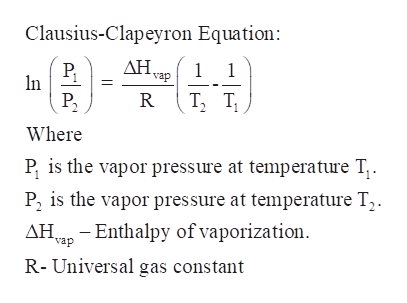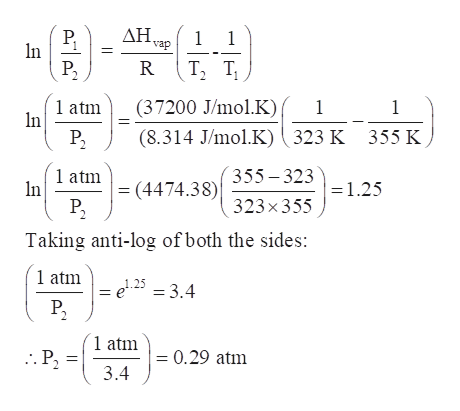# If the normal boiling point of a liquid is 82 °C, what is the vapor pressure (in atm) at 50 °C? Enthalpy of vaporization = 37.2 kJ/mol K.    A. 0.61 atmB. 0.73 atmC. 0.29 atmD. 0.11 atmE. 1.0 atm

Question
8 views

If the normal boiling point of a liquid is 82 °C, what is the vapor pressure (in atm) at 50 °C? Enthalpy of vaporization = 37.2 kJ/mol K.

A. 0.61 atm
B. 0.73 atm
C. 0.29 atm
D. 0.11 atm
E. 1.0 atm

check_circle

Step 1

If the normal boiling point of a liquid is 82 °C, the vapor pressure (in atm) at 50 °C is to be calculated.

Enthalpy of vaporization = 37.2 kJ/mol K.

Step 2

Clausius-Clapeyron equation: It is used to relate the vapor pressures of a substance at two different temperatures if the enthalpy of vaporization is known.help_outlineImage TranscriptioncloseClausius-Clapeyron Equation: P, In P, vap Т, т, R Where P, is the vapor pressure at temperature T,. P, is the vapor pressure at temperature T,. AHa - Enthalpy of vaporization. vap R- Universal gas constant fullscreen
Step 3

Given conditions are:

ΔHvap =37.2 kJ/mol.K = 37200 J/mol.K

T1 = 82°C (boiling point) = 82°C + 273 = 355 K

T2 = 50°C = 50°C +273 = 323 ...help_outlineImage TranscriptioncloseΔΗ. P, In P, vap Т, т, R 1 atm In (37200 J/mol.K) (8.314 J/mol.K)( 323 K P2 355 K 1 atm = (4474.38) 355- 323 In =1.25 P, 323x 355 Taking anti-log of both the sides: 1 atm = e25 = 3.4 P, ´1 atm :. P2 = 0.29 atm 3.4 fullscreen

### Want to see the full answer?

See Solution

#### Want to see this answer and more?

Solutions are written by subject experts who are available 24/7. Questions are typically answered within 1 hour.*

See Solution
*Response times may vary by subject and question.
Tagged in

### Physical Chemistry